# NUCLEAR EQUATIONS 1 Write a nuclear equation for

• Slides: 5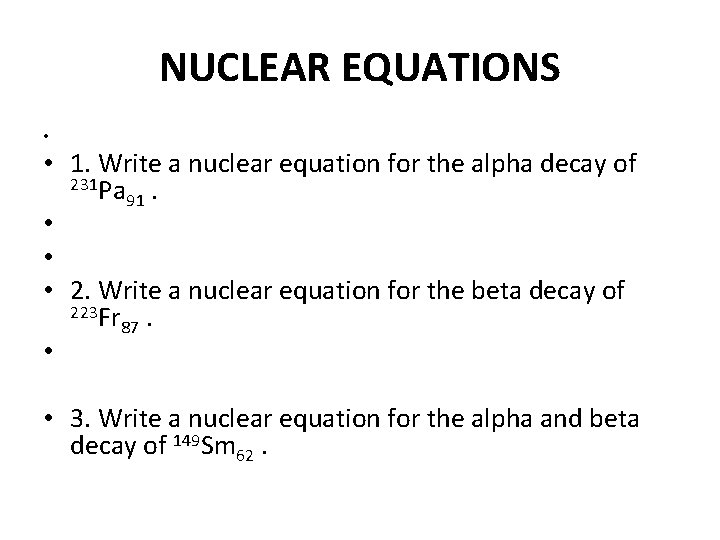NUCLEAR EQUATIONS • • 1. Write a nuclear equation for the alpha decay of 231 Pa . 91 • • • 2. Write a nuclear equation for the beta decay of 223 Fr . 87 • • 3. Write a nuclear equation for the alpha and beta decay of 149 Sm 62.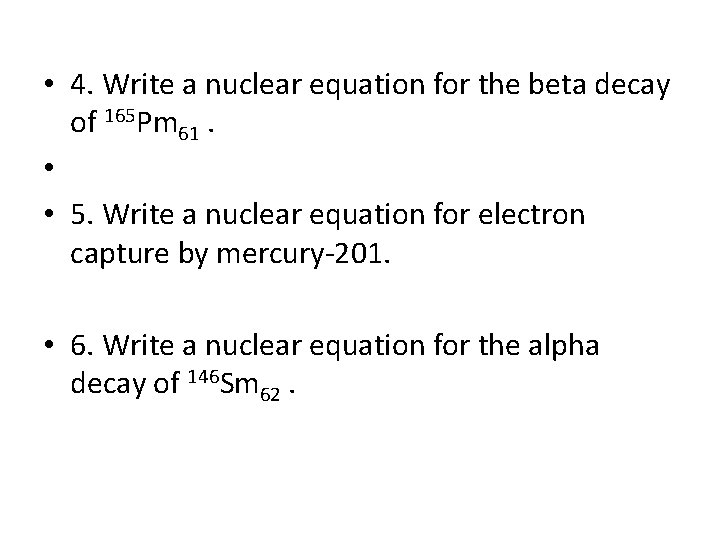• 4. Write a nuclear equation for the beta decay of 165 Pm 61. • • 5. Write a nuclear equation for electron capture by mercury-201. • 6. Write a nuclear equation for the alpha decay of 146 Sm 62.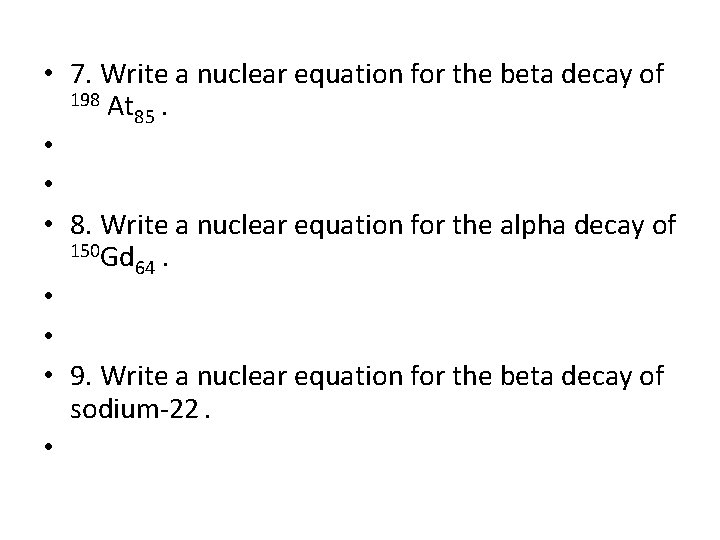• 7. Write a nuclear equation for the beta decay of 198 At . 85 • • • 8. Write a nuclear equation for the alpha decay of 150 Gd . 64 • • • 9. Write a nuclear equation for the beta decay of sodium-22. •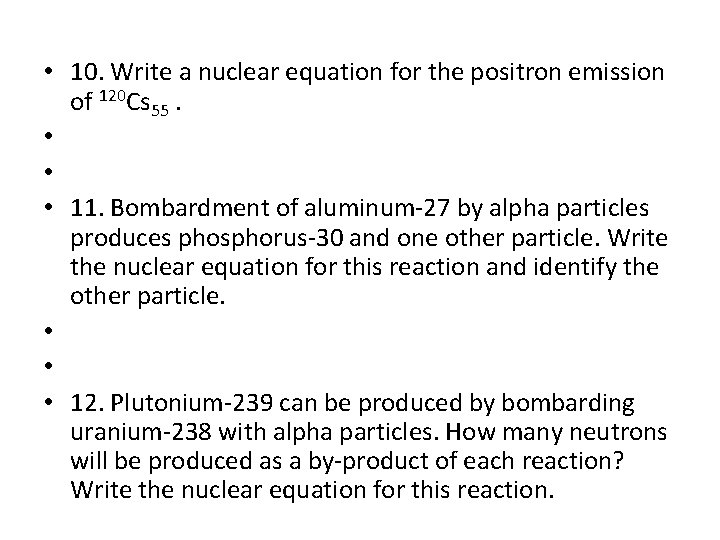• 10. Write a nuclear equation for the positron emission of 120 Cs 55. • • • 11. Bombardment of aluminum-27 by alpha particles produces phosphorus-30 and one other particle. Write the nuclear equation for this reaction and identify the other particle. • • • 12. Plutonium-239 can be produced by bombarding uranium-238 with alpha particles. How many neutrons will be produced as a by-product of each reaction? Write the nuclear equation for this reaction.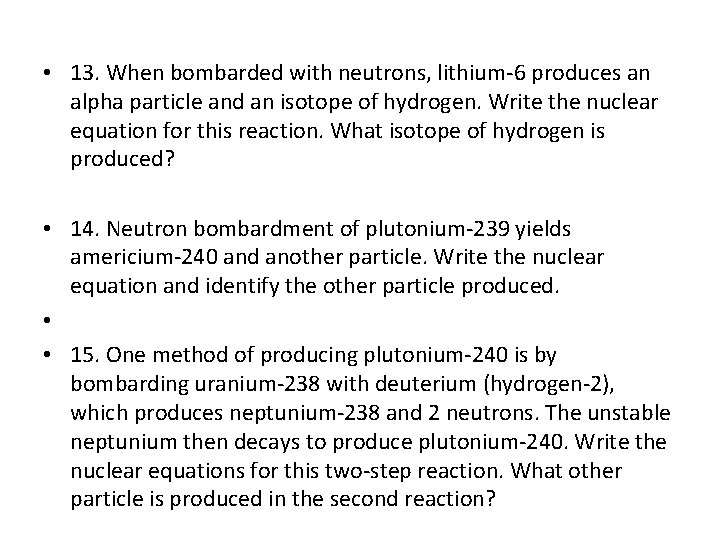• 13. When bombarded with neutrons, lithium-6 produces an alpha particle and an isotope of hydrogen. Write the nuclear equation for this reaction. What isotope of hydrogen is produced? • 14. Neutron bombardment of plutonium-239 yields americium-240 and another particle. Write the nuclear equation and identify the other particle produced. • • 15. One method of producing plutonium-240 is by bombarding uranium-238 with deuterium (hydrogen-2), which produces neptunium-238 and 2 neutrons. The unstable neptunium then decays to produce plutonium-240. Write the nuclear equations for this two-step reaction. What other particle is produced in the second reaction?